【数据结构与算法】第五章：散列

# 第五章：散列

• 散列表(hash table)：只支持二叉查找树所允许的一部分操作.
• 散列(hashing)：一种用于以常数平均时间执行插入,删除和查找的级数.

## 5.1 一般想法

• 理想的散列表数据结构只不过是一个包含有关键字的具体固定大小的数组.一般而言,这个关键字就是带有一个相关之的字符串.
• 我们把表的大小记作 TableSize T a b l e S i z e $TableSize$,并将其理解为散列数据结构的一部分而不仅仅是浮动于全局的某个变量.通常的习惯是让表从 0 0 $0$ TableSize1 T a b l e S i z e − 1 $TableSize - 1$ 变化.
• 散列函数(hash function)：每个关键字映射到从 0 0 $0$ TableSize1 T a b l e S i z e − 1 $TableSize - 1$ 这个范围中的某个数,并且被放到适当额单元中.最理想的情况是,运算简单并且任何两个不同的关键字映射到不同的单元.但是这是不可能的,因为单元的数目是有限的,而关键字是用不完的.
• 冲突(collision)：当两个关键字散列到同一个值的时候.

## 5.2 散列函数

• 当关键字是整数时,我们保证表的大小是一个素数,可以直接返回 KeymodTableSize K e y m o d T a b l e S i z e $Key mod TableSize$
• 当关键字是字符串时,散列函数需要仔细选择.
1. 一种选择方法是可以将字符串中的字符的 ASCII 码值相加起来.如下
//将字符逐个相加来处理整个字符串
typedef unsigned int Index;
Index Hash( const char *Key, int TableSize){
unsigned int HashVal = 0;
while( *Key != '\0')
HashVal += *Key++;
return HashVal % TableSize;
}

2.另一种散列函数如下

Index Hash2( const char *Key, int TableSize){
return( Key + 27 * Key + 729 * Key) % TableSize;
}

3.第三种散列函数

Index Hash3( const char *Key, int TableSize){
unsigned int HashVal = 0;
while( *Key != '\0')
HashVal = ( HashVal<<5) + *Key++;
return HashVal % TableSize;
}

## 5.3 解决冲突的方法

### 5.3.1 分离链接法

• 分离链接法(separate chaining)：将散列到同一个值的所有元素保留到一个表中.为了方便,这些表都有表头.如下图.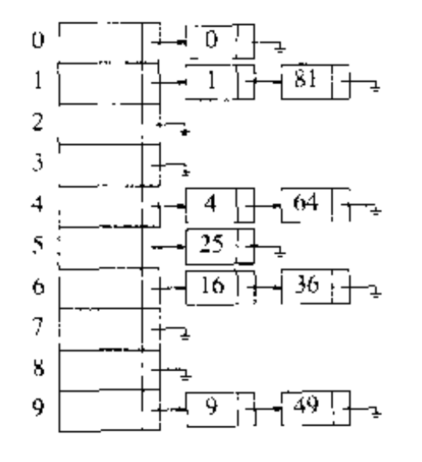1. Find操作,我们使用散列函数来确定究竟是哪一个表.此时,我们通常的方式遍历该表并返回所找到的被查找项所在的位置.
2. Insert操作,我们遍历整个表以检查该元素是否已经处在适当的位置.如果要插入重复元,那么通常要留出一个额外的域,这个域当重复元出现时候增加1.如果这个元素是个新元素,那么它或者被插入表的前端,或者被擦汗如到表的末尾.又是新元素插入到表的前端不仅是因为方便,而且新插入的元素可能最先被访问.
struct ListNode;
typedef struct ListNode *Position;
struct HashTbl;
typedef struct HashTbl *HashTable;

struct ListNode{
ElemeentType Element;
Position Next;
};

struct HashTbl{
int TableSize;
List *TheLists; //指向ListNode指针的指针
};

HashTable InitializeTable( int TableSize);
void DestroyTable( HashTable H);
Position Find( ElementType Key, HashTable H);
void Insert( ElementType Key, HashTable H);
ElementType Retrieve( Position P);
//初始化过程
HashTable InitializeTable( int TableSize){
HashTable H;
int i;

if( TableSize < MinTableSize){
Error(" Table is so small");
return NULL;
}

H = ( HashTable)malloc( sizeof( struct HashTbl));
if( H == NULL)
FatalError(" Out of space");

H->TableSize = NextPrime( TableSize);
H->TheLists = malloc( sizeof( List) * H->TableSize);
if( H->TheLists == NULL)
FatalError(" Out of space");

for( i=0; i<H->TableSize; i++){
H->TheList[i] = ( Position)malloc( sizeof( struct ListNode));
if( H->TheList[i] == NULL)
FatalError(" Out of space");
else
H->TheLists[i]->Next == NULL;
}
}

//寻找并返回一个指针
Position Find( ElementType Key, HashTable H){
Position P;
List L;
L = H->TheLists[ Hash( key, H->TableSize)];
P = L->Next;//L是头指针
while( P!=NULL && P->Element !=Key)
P = P->Next;
return P;
}

//插入
void Insert( ElementType Key, HashTable H){
Position Pos, NewCell;
List L;
Pos = Find( Key, H);
if( Pos == NULL){
NewCell = ( Position)malloc( sizeof( struct ListNode));
if( NewCell == NULL)
FatalError(" Out of space");
else{
L = H->TheLists[ Hash( Key, H->TableSize)];
NewCell->Next = L->Next;
NewCell->Element = Key; //当是整数时,Find同.
L->Next = NewCell;
}
}
}
• 分离链接散列算法的缺点

1. 需要指针.
2. 给新单元分配地址需要时间,导致了算法时间缓慢.
• 装填因子(load factor) λ λ $\lambda$：散列表中元素的个数与散列表大小的比值.

### 5.3.2 开放定址法

• 开放定址散列法( Open addressing hashing)：如果有冲突发生,那么就要尝试选择另外的单元,直到找出空的单元为止.更一般地,单元 h0(X),h1(X),h2(X), h 0 ( X ) , h 1 ( X ) , h 2 ( X ) , $h_0(X), h_1(X), h_2(X),$ 等等相继被试选其中 hi(X)=(Hash(X)+F(i))modTableSize h i ( X ) = ( H a s h ( X ) + F ( i ) ) m o d T a b l e S i z e $h_i(X) = ( Hash(X) + F(i)) mod TableSize$, 且 F(0)=0 F ( 0 ) = 0 $F(0) = 0$ .
考虑到所有的数据都要置入表内部,因此开放定址散列法需要的表比分离链接散列表的表要大.一般而言, 装填因子 λ λ $\lambda$ 应该低于 0.5 .

#### 5.3.2.1 线性探测法

• 在线性探测法中,函数 F F $F$ i i $i$ 的线性函数,典型情形是 F(i)=i F ( i ) = i $F(i) = i$ .这相当于逐个探测每个单元以查找出一个空单元.
for example: 把关键字{ 89, 18, 49, 58, 69},插入到一个散列表中,此时的冲突解决办法选择 F(i)=i F ( i ) = i $F(i) = i$ .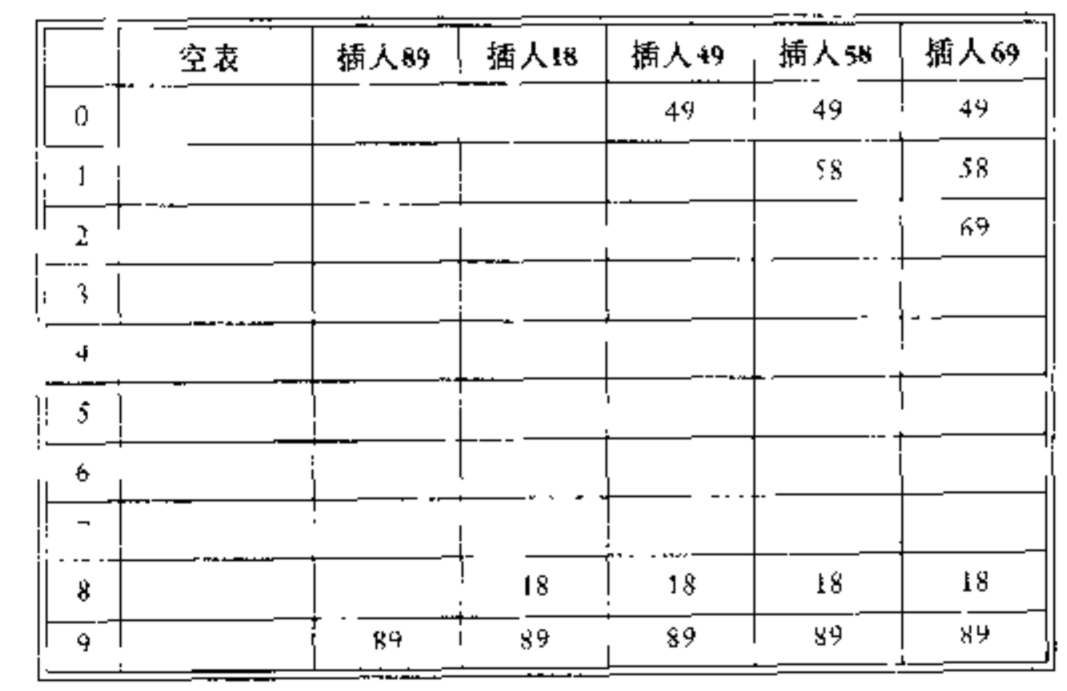第一个冲突发生在插入关键字 49 时,它被放到下一个空闲地址,即 0 处.
随后,关键字 58 依次与 18,89,49 发生冲突,试选三次后才找到空单元 2 .
关键字 69 亦是如此.
只要表足够大,我们总能找到一个自由单元,但是花费的时间是非常多的.

• 一次聚集(primary clustering)：使用线性探测法时,即时表相对于比较空的时候,一些占据的单元也会形成一些区块.

#### 5.3.2.2 平方探测法

• 平方探测发时消除线性探测中狙击问题的冲突解决方法.本质上就是冲突函数为幂次函数的探测方法.流行的选择是 F(i)=i2 F ( i ) = i 2 $F(i) = i^2$ .
for example: 把关键字{ 89, 18, 49, 58, 69},插入到一个散列表中.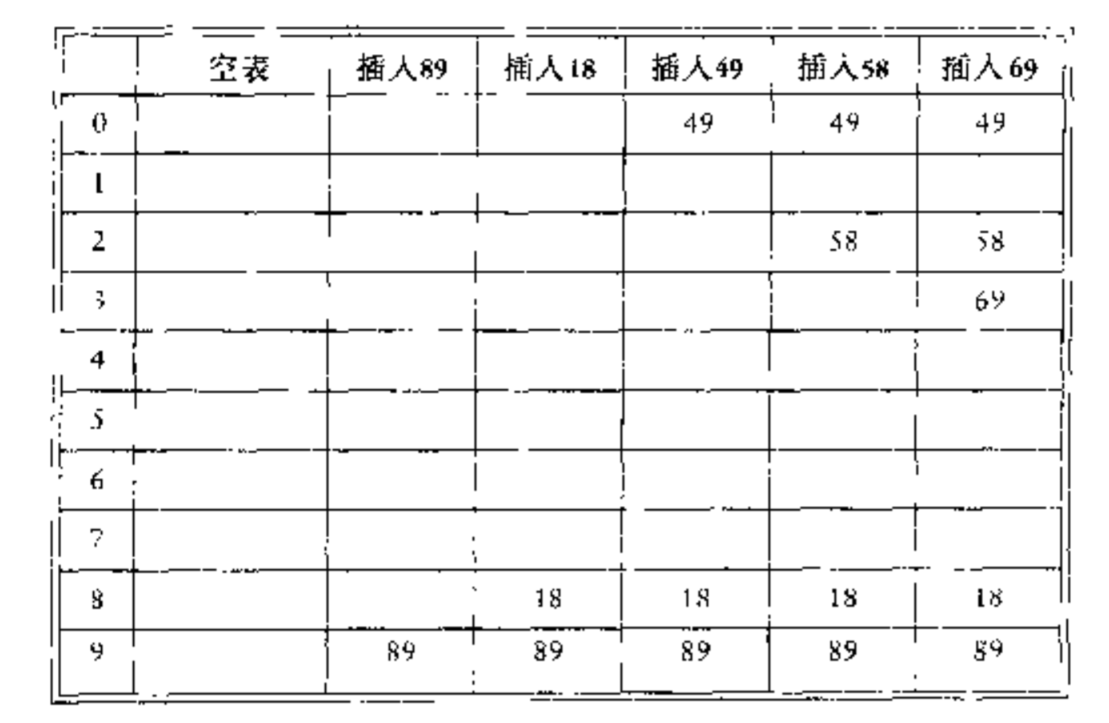当 49 与 89 冲突时,下一个位置为下一个单元,该单元是空的,因此 49 就放在此处.
此后 58 在位置 8 处发生冲突,其后相邻的单元经过探测得知发生了另外的冲突.下一个探测的位置就在距离位置 8 为 2^2 = 4 远处,此时,这个单元也是空单元,因此 关键字 58 就放在单元 2 的位置处.
对于关键字 69 处理的过程也是一样.

• 对于线性探测,让元素几乎填满散列表并不是个好主意,因为这时候表的性能将会降低.

• 对于平方探测,一旦表被填写超过一半,当表的大小不是素数甚至在表被填满一半之前,就不能保证可以找到一个空单元了.这是因为最多有表的一般可以用作解决冲突的备选位置.

• 定理
如果使用平方探测,并且表的大小是素数,那么当表至少有一半是空的时候,总能够插入一个新的元素.

h(X)+i2=h(X)+j2(modTableSize) h ( X ) + i 2 = h ( X ) + j 2 ( m o d T a b l e S i z e ) $h(X) + i^2 = h(X) + j^2 (mod TableSize)$
i2=j2(modTableSize) i 2 = j 2 ( m o d T a b l e S i z e ) $i^2 = j^2 (mod TableSize)$
(ij)(i+j)=0(modTableSize) ( i − j ) ( i + j ) = 0 ( m o d T a b l e S i z e ) $( i - j)( i + j) = 0 (mod TableSize)$

typedef unsigned int Index;
typedef Index Position;

struct HashTabl;
typedef struct HashTbl * HashTable;

struct HashEntry{
ElementType      Element;
Enum KindOfEntry Info;
};

typedef struct HashEntry Cell;

struct HashTbl{
int TableSize;
Cell *TheCells;
};

HashTable InitializeTable( int TableSize);
void DestroyTable( HashTable H);
Position Find( ElementType Key, HashTable H);
void Insert( ElementType Key, HashTable H);
//初始化开放定址散列表
HashTable InitializeTable( int TableSize){
HashTable H;
int i;
if( TableSize < MinTableSize){
Error(" Table size is too small");
return NULL;
}

H = ( HashTable)malloc( sizeof( struct( HashTbl)));
if( H == NULL)
FatalError(" Out of space");

H->TableSize = NextPrime( TableSize);
H->TheCells = malloc( sizeof( Cell) * H->TableSize);
if( H->TheCells == NULL)
FatalError(" Out of space");

for( i = 0; i < H->TableSize; i++)
H->TheCells[i].Info = Empty;

return H;
}
//Find
Position Find( ElementType Key, HashTable H){
Position CurrentPos;
int CollisionNUm;

CollisionNum = 0;
CurrentPos = Hash( Key, H->TableSize);
while( H->TheCells[ CurrentPos].Info != Empty &&
H->TheCells[ CurrentPos].Element != Key){
CurrentPos += 2 * ++CollisionNum - 1;
if( CurrentPos >= H->TableSize )
CurrentPos-= H>TableSize;
}
return CurrentPos;
}
//Insert
void Insert( ElementType Key, HashTable H){
Position Pos;
Pos = Find( Key, H)
if( H->TheCells[ Pos].Info != Legitimate){
H->TheCells[ Pos].Info = Legitimate;
H->TheCells[ Pos].Element = Key;
}
}
• 二次聚集(secondary clustering)

#### 5.3.2.3 双散列

• 双散列(double hashing)：对于双散列,一种流行的选择是 F(i)=iHash2(X) F ( i ) = i ∗ H a s h 2 ( X ) $F(i) = i * Hash_2(X)$ 这个公式意味着,我们将第二个散列函数应用到 X X $X$ 并在距离 Hash2(X),2Hash2(X) H a s h 2 ( X ) , 2 H a s h 2 ( X ) $Hash_2(X) , 2Hash_2(X)$ 等处探测.但是要记住, Hash2(X) H a s h 2 ( X ) $Hash_2(X)$ 的选择不好将要导致灾难性的后果.
for example：把 99 插入到 { 89, 18, 49, 58, 69} 中.通常选择的是 Hash2(X)=Xmod9 H a s h 2 ( X ) = X m o d 9 $Hash_2(X) = X mod 9$ 将不再起作用.

## 5.4 再散列

• 对于使用平方探测的开放定址散列法,如果表的元素被填充的太满,那么操作的运行时间将会开始消耗的非常场,并且 Insert 操作可能失败.这种情况可能发生在有太多插入和移动的情形下.
• 一种解决办法：建立另外一个大约两倍大的表(而且使用一个相关的新散列函数),扫描整个源是散列表,计算每个未删除的元素的新散列值并将它插入到新的散列表中.
for example：将元素 13, 15, 24, 6插入到大小为 7 的开放定制散列表中.其中散列函数是 h(X)=Xmod7 h ( X ) = X m o d 7 $h(X) = X mod 7$.假设使用线性探测法解决这个问题,那么插入结果如下图所示.
06
115
2
324
4
5
613

06
115
223
324
4
5
613

0
1
2
3
4
5
66
723
824
9
10
11
12
1313
14
1515
16
17

- 以上这个过程就叫做再散列(rehashing).但我们可以观察到这是一个非常昂过的操作;其运行时间是 O(N) O ( N ) $O(N)$ 因为有 N N $N$ 个元素要再散列得到的表大小约为 2N 2 N $2N$.不过,由于不经常发生,因此实际效果根本没有这么差.特别地,在最后的再散列之前必然已经存在 N2 N 2 $\frac{N}{2}$ 次 Insert 操作，当然添加到每一个插入上的花费基本是一个常数开销.
- 再散列可以用平方探测以多种方法实现.
1. 一种做法是只要表满到一半就再散列.
2. 一种极端的方法是只有插入失败的时候再再散列.

 HashTable Rehash( HashTable H){
int i,OldSize;
Cell *OldCells;

OldCells = H->TheCells;
OldSize = H->TableSize;

H = InitializeTable( 2 * OldSize);

for( i = 0; i <= OldSize; i++){
if( OldCells[i].Info == Legitimate)
Insert( OldCells[i].Element, H);
}
free(OldCells)
return H;
}

## 5.5 可扩散列

• 当数据量太大以至于装不进主存时,此时主要考虑的时检索数据所需要的硬盘存取次数.
• 我们假设任意时刻都有 N N $N$ 个记录需要储存, N N $N$ 的值伴随时间变化而发生改变. 此外,最多可以把 M M $M$ 个记录放入一个磁盘区块.
如果使用开放定址散列法或者分离链接散列法,主要问题在于一次 Find 操作期间冲突可能引起多个区块被考察,甚至对于理想分布的散列表亦是如此.不仅如此,当表过满的时候,必须执行代价巨大的再散列操作,它需要 O(N) O ( N ) $O(N)$ 次磁盘访问.
• 可扩散列(extendible hashing)：它允许用两次磁盘访问执行一次 Find 操作.插入操作也需要很少的磁盘访问.
• 现在我们假设数据由几个 6 比特的整数组成,参考下图,我们使用B-树的形式储存.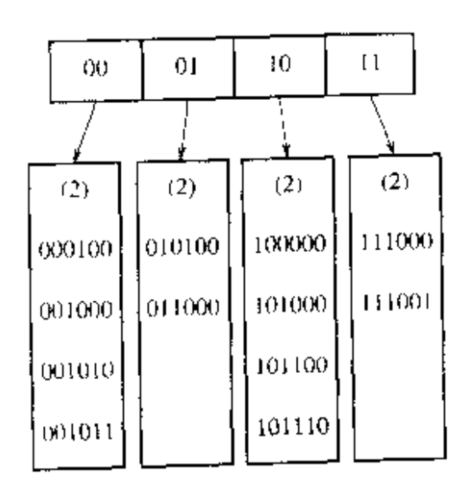“树”根含有 4 个指针,它们由这些数据的前两个比特来确定.每片树叶之多有 M=4 M = 4 $M = 4$ 个元素.碰巧的时,这里没一片树叶中的数据前两个比特都是相同的.为了更正,我们用 D D $D$ 表示根所使用的比特数,可称之为 目录(directory).于是,目录中的项数为 2D 2 D $2^D$. dL d L $d_L$ 是树叶 L L $L$所有元素共有的最高位的位数, dLD d L ≤ D $d_L \le D$.
现在想要插入关键字 100100 ,它进入第三片树叶,但是第三片树叶已经满了,没有空间存放它,此时我们将这片树叶分裂成两片树叶,它们由前三个比特确定.这需要将目录的大小增加到 3.如下图.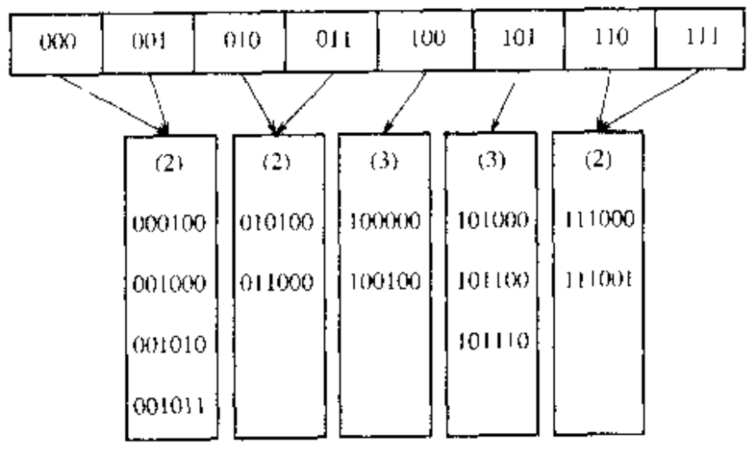注意,所有未被分裂的树叶现在各由两个相邻的目录所指.因此,虽然整个目录被重写,但是其他树叶实际上并没有被访问.
现在如果插入关键字 000000 ,那么第一片树叶就要被分裂,生成 dL=3 d L = 3 $d_L = 3$ 的两片树叶,由于 D=3 D = 3 $D = 3$ ,因此再目录中唯一的变化便是 000 和 001 指针的更新.如下图.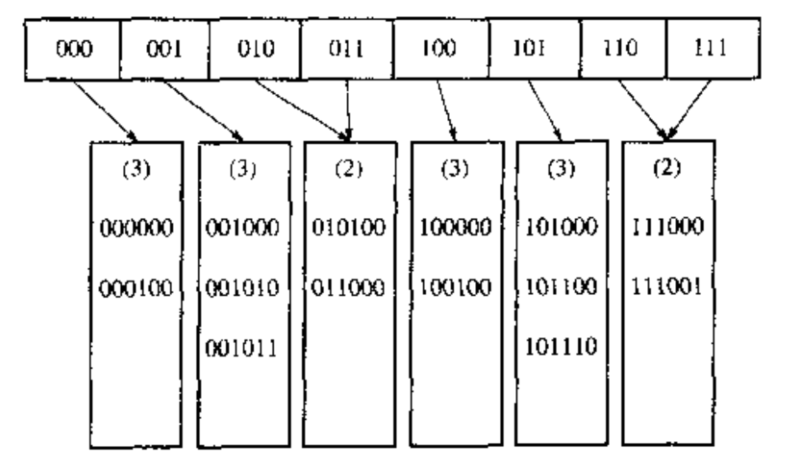• 这里我们还有一些重要的细节尚未考虑.
首先,有可能当一片树叶的元素多余 D+1 D + 1 $D + 1$ 个前导位相同时需要多个目录分裂.例如,从原先的例子开始看起, D=2 D = 2 $D = 2$ ,如果插入 111010, 111011 并在最后插入 111100,那么目录的大小必须增加到 4 以区分这五个关键字.
其次,存在重复关键字(duplicate key)的可能性,若存在多余 M M $M$ 个重复关键字,那么该算法根本无效,需要做出其他安排.

• 可扩散列的性能：
一个合理的假设：位模式(bit pattern)是均匀分布的.
基于这个假设,我们得到树叶的期望个数为 NMlog2e N M l o g 2 e $\frac{N}{M}log_2e$.因此,平均树叶满的程度为 ln2 l n 2 $ln2$.这个和B-树是一样的,这并不奇怪,因为对于这两个数据结构而言,当第 M+1 M + 1 $M + 1$ 被添加时,一些新的节点便会将建立起来.
目录的期望大小为 O(N1+1/M/M) O ( N 1 + 1 / M / M ) $O(N^{1+1/M}/M)$ 因此,如果 M M $M$ 的值很小,那么目录可能过分地大.为了位置更小的目录,可以把第二个磁盘访问添加到每个 Find 操作中去.如果目录太大装不进主存,那么第二个磁盘访问怎么说还是需要的.

## 我的微信公众号08-2121901-221552
02-265550
10-041513
08-241万+
10-3163
12-24642
08-051万+
06-121260
04-0673